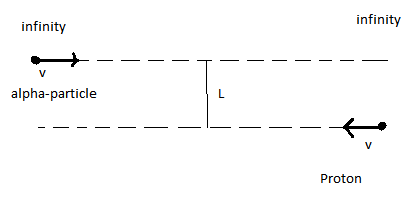# Distance of closest approachA proton and an $\alpha$-particle are projected with velocity $v =\sqrt { \frac { { e }^{ 2 } }{ 4\pi { \varepsilon }_{ 0 }mL } }$ each from infinity as shown.The perpendicular distance between their initial velocities is $L$.Let the distance of their closest approach be $D$.

Given $x={ (\frac { 8D }{ L } -5) }^{ 2 }$.Find $x$

Details and assumptions

1)mass of proton=$m$,charge=$+e$

2)mass of $\alpha$-particle=$4m$,charge=$+2e$

3)${ \varepsilon }_{ 0 }$=Vacuum Permittivity

×